Resistors in Series and Parallel

• Last Updated : 28 Sep, 2021

While studying the electric current, we usually describe resistance as an obstruction in the path of current. Resistors are the devices that offer resistance to the current. In our daily lives, our devices have more than one resistance in the circuits. It becomes essential to study the effect of different arrangements of resistances and their effects on the circuit. Often in real situations, it is required to calculate the required resistance for a full circuit or sometimes a portion of the circuit. In such cases, the knowledge of calculating equivalent resistances can be useful, let’s see these concepts in detail.

Resistors and Resistance

Resistors are electrical devices that restrict the flow of current in a circuit. It is an ohmic device, which means that it follows the ohms’ law V = IR. Most of the circuits have only one resistor, but sometimes more than one resistor can be present in the circuit. In that case, the current flowing through the circuit depends on the equivalent resistance of the combination. These combinations can be arbitrarily complex, but they can be divided into two basic types:

1. Series Combination
2. Parallel Combination

Series Combination

In the figure given below, three resistors are connected in series with the battery of voltage V. In this type of combination, resistors are usually connected in a sequential manner one after another. The current through each resistor is the same. The figure on the right side shows the equivalent resistance of the three resistances. In the case of the series combination of resistances, the equivalent resistance is given by the algebraic sum of the individual resistances.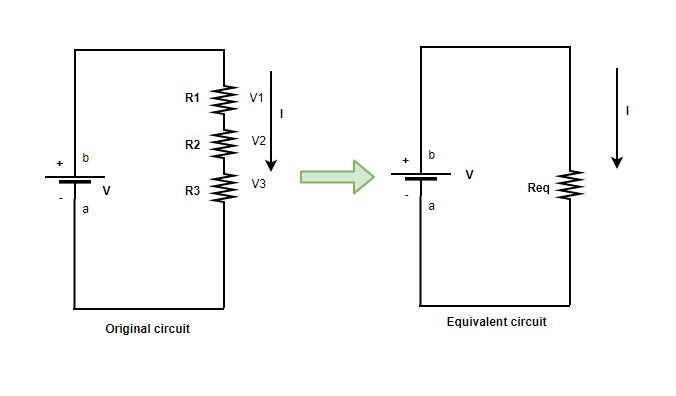Let V1, V2, and V3 be the voltages across all three resistances. It is known that the current flowing through them is the same.

V = V + V2 + V3

Expanding the equation,

IR = IR + IR2 + IR3

R = R1 + R2 + R3

Parallel Combination

In the figure given below, three resistors are shown which are connected in parallel with a battery of voltage V. In this type of connection, the resistors are usually connected on parallel wires originating from a common point. In this case, the voltage through each resistor is the same. The figure on the right side shows the equivalent resistance of the three resistances.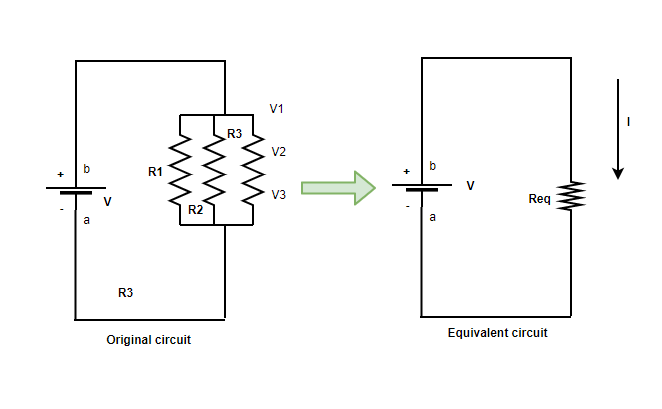The relation between voltage and current is given by,

V = IR

It can be rewritten as,

I = V/R

The voltages across individual resistors will be,

I1 = V/R1 , I2 = V/R2, I3 = V/R3

The total current across all the resistances will be,

I = I1 + I2 + I3

Substituting the expressions for individual voltages,

I = V/R1 + V/R + V/R3

Let the equivalent capacitance be R,

V/R = V/R1 +V/R + V/R3

Upon Simplifying the above equation, the relation becomes,In general for capacitors R1, R2, R3, ….Sample Problems

Question 1: Three resistances of 3, 5, and 10 ohms are connected in series. Find the equivalent resistance for the system.

The formula for series resistance is given by,

R = R1 + R2 + R3

Given: R1 = 3, R = 5 and R3 = 10

Substituting these values in the equation,

R = R1 + R2 + R3

⇒ R = 3 + 5 + 10

⇒ R = 18 Ω

Question 2: Three resistances of 2, 2, and 4 ohms are connected in parallel. Find the equivalent resistance for the system.

The formula for parallel resistance is given by,Given: R1 = 2, R = 2 and R3 = 4

Substituting these values in the equation,⇒⇒⇒Ω

Question 3: Find the equivalent resistance for the system shown in the figure below.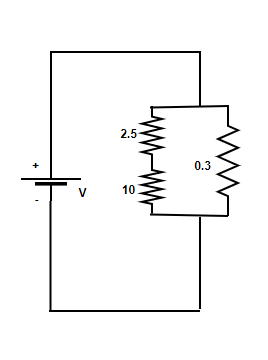The formula for parallel resistance is given by,And the formula for series resistance is given by,

R = R1 + R2 + R + ….

This is combination of both parallel and series resistances.

Substituting these values in the equation,

R1 = 10 Ω, R2  = 2.5 Ω

R= R1 + R2

⇒ R = 10 + 2.5

⇒ R = 12.5⇒⇒⇒Ω

Question 4: Find the equivalent resistance for the system shown in the figure below.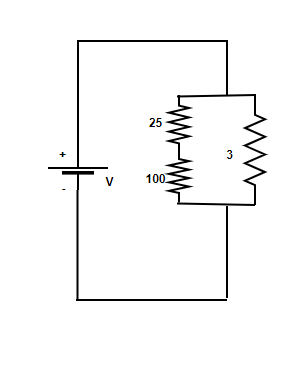The formula for parallel resistance is given by,And the formula for series resistance is given by,

R = R1 + R2 + R + ….

This is combination of both parallel and series resistances.

Substituting these values in the equation,

R1 = 100 Ω, R2 = 25 Ω

R= R1 + R2

⇒ R = 100 + 25

⇒ R = 125⇒⇒⇒Ω

Question 5: Find the equivalent resistance for the system shown in the figure below.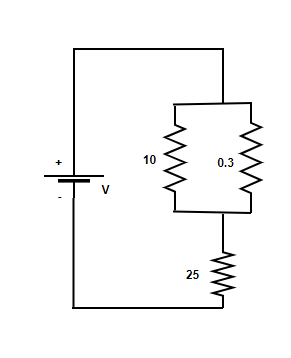The formula for parallel resistance is given by,And the formula for series resistance is given by,

R = R1 + R2 + R + …

This is combination of both parallel and series resistances.

Substituting these values in the equation,

R1 = 10 Ω, R2  = 0.3 Ω⇒⇒⇒⇒ R = 0.29

R = R1 + R2

⇒ R = 0.29 + 25

⇒ R = 25.29Ω

My Personal Notes arrow_drop_up